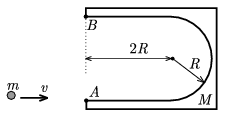Mathematical and Physical Journal
for High Schools
Issued by the MATFUND Foundation
 Already signed up? New to KöMaL?

#Problem P. 3938. (December 2006)

P. 3938. The U-shaped body of mass M shown in the figure is at rest and lies on a smooth horizontal surface (e.g. on ice). Its centre of mass is the midpoint of the semicircle of radius R. The length of the straight arms of the U is 2R. A small disc of mass m slides tangentially to point A on one of the arms, with a velocity of v.a) Where will point A be when the disc is at point B on the other arm?

b) Draw the path of the two bodies.

c) Find the velocity versus time and acceleration versus time functions for both bodies.

(Friction and air drag are negligible everywhere. Data: M=m=1 kg, R=0.1 m, v=0.5 m/s.)

(5 pont)

Deadline expired on January 11, 2007.

### Statistics:

 17 students sent a solution. 5 points: Nyíri Dávid Ákos, Szolnoki Lénárd, Varga Bonbien. 4 points: Almási 270 Gábor András, Almasi Susann Melinda, Csengeri Béla, Farkas Ádám László, Roósz Gergő, Tüzes Dániel. 3 points: 4 students. 2 points: 3 students. 1 point: 1 student.

Problems in Physics of KöMaL, December 2006